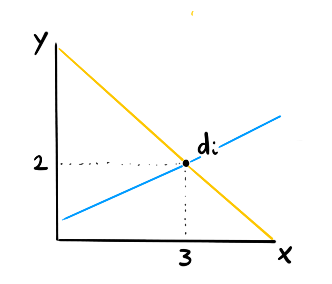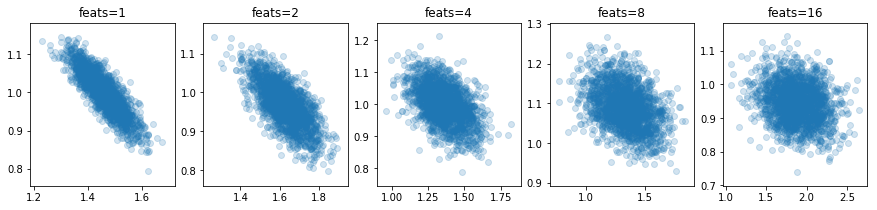# Theoretical Dependence

The simplest regression might look something like this;

$\hat{y}_i \approx \text{intercept} + \text{slope } x_i$

You might assume at this point in time that the slope and intercept are unrelated to eachother. In econometrics you're even taught that this assumption is necessary. If you're one I have to warn you for what you're about to read. I'm about to show you why, by and large, this independance assumption is just plain weird.

## Posterior

Let's generate some fake data and let's toss it in PyMC3.

import numpy as np
import matplotlib.pylab as plt

n = 1000
xs = np.random.uniform(0, 2, (n, ))
ys = 1.5 + 4.5 * xs + np.random.normal(0, 1, (n,))


## PyMC3

Let's now throw this data into pymc3.

import pymc3 as pm

with pm.Model() as model:
intercept = pm.Normal("intercept", 0, 1)
slope = pm.Normal("slope", 0, 1)
values = pm.Normal("y", intercept + slope * xs, 1, observed=ys)
trace = pm.sample(2000, chains=1)

plt.scatter(trace.get_values('intercept'), trace.get_values('slope'), alpha=0.2)
plt.title("pymc3 results");


That's interesting; the intercept and slope variables aren't independant at all. They are negatively related!

## Scikit-Learn

Don't trust this result? Let's sample subsets and throw it into scikit learn, let's see what comes out of that.

size_subset = 500
n_samples = 2000
samples = np.zeros((n_samples, 2))

for i in range(n_samples):
idx = np.random.choice(np.arange(n), size=size_subset, replace=False)
X = xs[idx].reshape(n_samples, 1)
Y = ys[idx]
sk_model = LinearRegression().fit(X, Y)
samples[i, 0] = sk_model.intercept_
samples[i, 1] = sk_model.coef_

plt.scatter(samples[:, 0], samples[:, 1], alpha=0.2)
plt.title("sklearn subsets result");


## Why?

So what is going on here? We generated the data with two independent parameters. Why are these posteriors suggesting that there is a relationship between the intercept and slope?

There are two arguments to make this intuitive.

### Argument One: Geometry

Consider these two regression lines that go into a single point.As far as the little point is concerned both lines are equal. They both have the same fit. We're able to exchange a little bit of the intercept with a little bit of the slope. Granted, this is for a single point, but also for a collection of points you can make an argument that you can exchange the intercept for the slope. This is why there must be a negative correlation.When the intercept is low, the slope must be high to fit a single point.

### Argument Two: A bit Causal

Consider this causal graph.

The $$x_0$$ node and $$x_1$$ node are independant, that is, unless $$y$$ is given. That is because, once $$y_i$$ is known, we're back to a single point and then the geometry argument kicks in. But also because logically we could explain the point $$y_i$$ in many ways; a lack of $$x_0$$ can be explained by an increase of $$x_1$$, vise versa or something in between. This encoded exactly in the graphical structure.

## Conclusion

It actually took me a long time to come to grips with this. Upfront the linear regression does look like the addition of independant features. But since they all need to sum up to a number, it is only logical that they are related.

Assuming properties of your model upfront is best done via a prior, not by an independence assumption.

## Appendix

The interesting thing about this phenomenon is that it is so pronounced in the simplest example. It is far less pronounced in large regressions with many features, like;

$$y_i \approx \text{intercept} + \beta_1 x_{1i} + ... + \beta_f x_{fi}$$ Here's some plots of the intercept value vs. the first estimated feature, $$\beta_1$$ given $$f$$ features;You can clearly see the covariance decrease as $$f$$ increases. The code for this can be found here.